Bascetta Star
 To the Main Page     "Mathematische Basteleien"

What is the Bascetta-Star?
 ...... The Bascetta Star is a three-dimensional star that is assembled from 30 modules.  The modules are folded from squares. Mathematically, it is an icosahedron with 20 triangular pyramids on its sides.
The name goes back to the inventor of the module and the star, the Italian Paolo Bascetta.

Comparison with the Great Stellated Dodecahedron  top
The great stellated dodecahedron has the same structure. It is also an icosahedron with straight triangular pyramids as the crown, but the pyramids are more pointed.Ikosaeder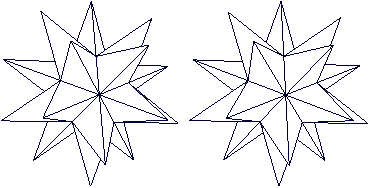...... The name dodecahedron is explained by the fact that a dodecahedron is formed, when you connect the vertices. The image pairs become three-dimensional with the 3D view.

 ...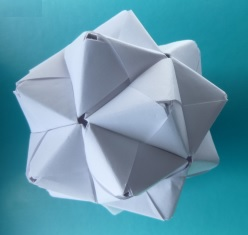... The Bascetta star is related to the Sonobe star, which is also a stellated icosahedron and which is also assembled from 30 modules.

Folding a Module     top
 ...... You need 30 squares of paper for the star. You can use the 9cm x 9cm note paper.

11 steps
01
 ...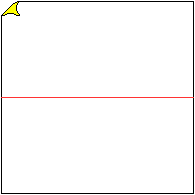... Halve the square with a valley fold.  It is convenient that for this fold and the following ones you do not just go over the crease with your finger, but, for example, with a pair of scissors inverted.02
 ...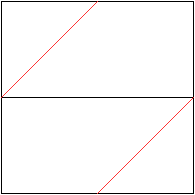... Fold on the red lines.03
 ...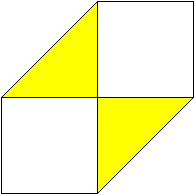... It should look like this.04
 ...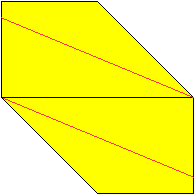... Turn the paper over and fold along the red lines.05
 ...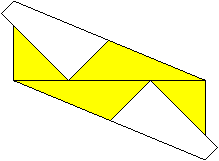It should look like this.06
 ...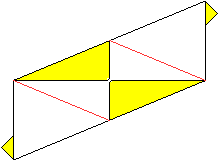... Turn the paper over and fold along the red lines.07
 ...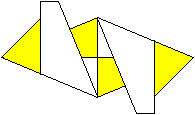... It should look like this.08
 ...... Turn the paper over and fold along the red lines.09
 ...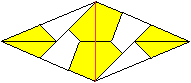... It should look like this. Fold along the red lines.10
 ...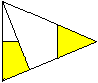..................... It should look like this.11
 ...... Unfold the triangle several times until you get back to figure 05.

 ...... You have to go through it. Fold the module 29 more times.

 ...... It should be noted that each module contributes to two faces of adjacent pyramids.

Joining to Form a Pyramid     top
 ...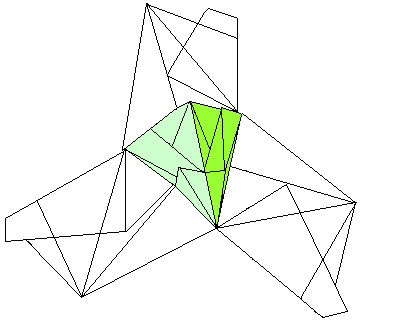. Three modules are plugged together to form a pyramid.

And it goes like this.
 ...... Put one module into the (pink) pocket of a second module.

 ...... It should look like this.

 ...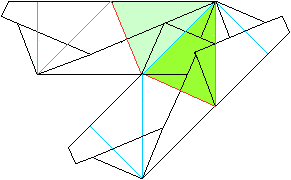... This is already two side faces of a pyramid.  The upper face is folded backwards.A third module encloses the first two and is fixed. The first pyramid is finished.

Assembling the Star   top
Modules, each forming another pyramid, are added to the first pyramid one after the other until the star finally closes.
 ...... It is a great help in the resulting confusion if you imagine the generating icosahedron:  Five triangles meet at each vertex.  This means that five pyramids always form a wreath.

Some Mathematics  top
 ...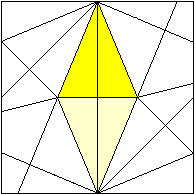... If you unfold the module, you will recognise two side faces of pyramids as isosceles triangles. The angle at the top of one triangle is 22.5° because of the folding process. The height of the triangle is half the side length of the square.

The angle 22.5° also occurs in the basic triangle of the regular octagon.
 ...... Therefore, the following formulas can be adopted. If the base  a is given, then the height in the triangle is r = (1/2)[sqrt(2)+1]a (rounded r = 1.207a)  and the leg is R = (1/2)sqrt[4+2sqrt(2)]a (rounded R = 1.307a).

Question 1
Only a small part of the square area A of the module is used. What percentage is this?

The two isosceles triangles together have the area 2A = ar.
The square of the module has the area A = 4r².
Then (2A)/A = (ar)/(4r²) = a/(4r) = 1/[2(sqrt(2)+1)] = (1/2)[sqrt(2)-1] = 0.207 = 20.7%.
Result
About 20% of the area of the initial square is used.

Question 2
What is the height H of a pyramid?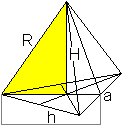According to the Pythagorean theorem  H² = (1/4)[4+2sqrt(2)]a²-[(2/3)(1/2)sqrt(3)]²a²  = [1+(1/2)sqrt(2)-1/3]a² = [2/3+(1/2)sqrt(2)]a² = (1/36)[24+18sqrt(2)]a² Then H = (1/6)sqrt[24+18sqrt(2)]a (rounded H = 1,172a)
Result
The height of a pyramid is H = (1/6)sqrt[24+18sqrt(2)]a (rounded 1.172a).

According to the website of wikipedia, the height of the pyramid of the Great Stellated Dodecahedron is
k = (1/6)[3sqrt(3)+sqrt(15)] = 1.516a, so it is larger than the pyramid of the Bascetta star.

Question 3
How big is the star?
This refers to the distance e between the tips of two opposite pyramids.

> The distance between opposite sides of the icosahedron is d=(1/6)[3sqrt(3)+sqrt(15)]a =1.152a.
> The height of a pyramid is H = (1/6)sqrt[24+18sqrt(2)]a = 1.172a.
Then e = 2H+d = (1/3)sqrt[24+18sqrt(2)]a+(1/6)[3sqrt(3)+sqrt(15)]a (rounded e = 3.496a).
It is convenient to relate the distance e to the side length 2r of the initial square.
It is r = 1.207a or a = 0.829r. Then e = 3.496a = 3.496*0.829r = 2.898r = 1.450(2r).
With 2r = 9cm, e = 13.04cm.
Results
If the side length of the initial square is 9cm, the star will be 13.04cm.
The ratio e/(2r) is 1.45. The star is about 50% bigger than the side length of the square.

More Figures from the Modules    top
Rudolf Kunstmann, who suggested this website, has obviously worked intensively with solids from the Bascetta modules and their variants.
With his permission I show a small selection of his stars.

Stellated octahedron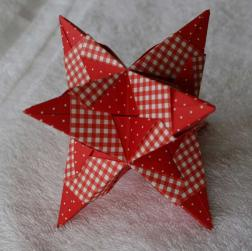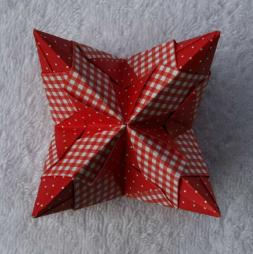Star to the cubeoctrahedron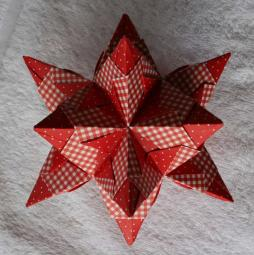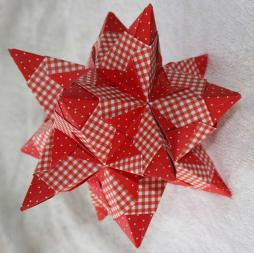Star to the rhombicuboctahedron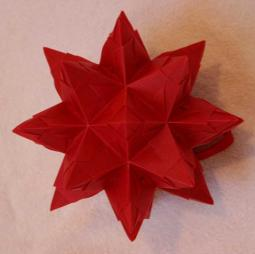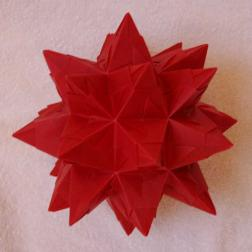Star to the snub cube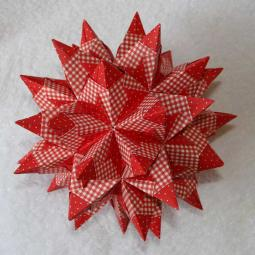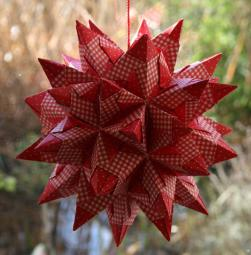Figure from other Modules        top
On my website Körper flechten I use another module to build a cube.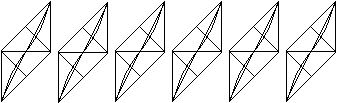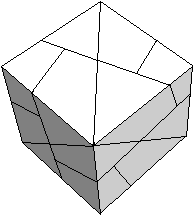Modular origami is a broad field on which you can find a lot in literature and on the internet.

Stellated Polyhedra     top
Stellated polyhedra are convex polyhedra with straight pyramids on their sides.
Here are a list of solids of this type that can be found on my homepage (German only).

Bekröntes Tetraeder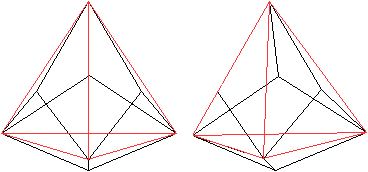Triakis-Tetraeder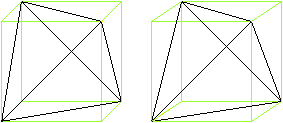Tetraeder im Würfel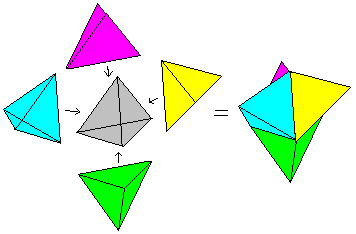Hypertetraeder

Bekröntes OktaederOktaedersternTriakisoktaeder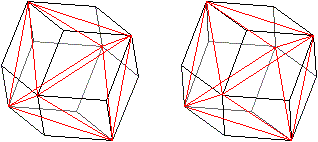...
Rhombendodekaeder

Bekrönter WürfelTetrakishexaederRhombendodekaeder

Bekröntes Ikosaeder und bekröntes PentagondodekaederGroßes Sterndodekaeder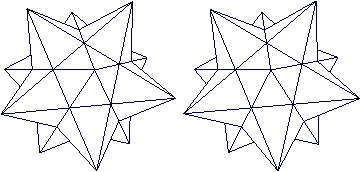Kleines Sterndodekaeder

Bekröntes RhombendodekaederYoshimoto Cube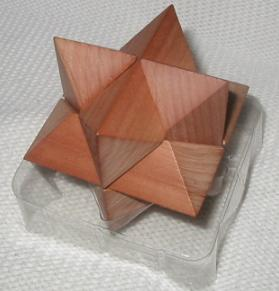Stern-Puzzle

Bekröntes Kleines Rhombenkuboktaeder
 ........ If you crowns the rhombicuboctahedron, the result is a star made of 18 square pyramids  and eight triangular ones. This is the shape of the well-known Herrnhut Stern or Moravian Star.

 ...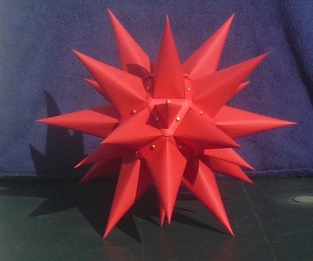This is the Herrnhut Star, built from a kit  (12/2013).

There is now (05/2022) another web page on a stellated icosahedron made of 20 squares, the Kusudama star.

Comparison of three stars
 a=9,0cm Sonobe Star Bascetta Star Kusudama Star 2A/Aq 12,7% 20,0% 26,8% H 0,29a = 2,6cm 0,49a = 4,4cm 0,70a = 6,3cm e 1,04a = 9,4 cm 1,45a = 13,1cm 1,97a = 17,7cm

 Sonobe Star Bascetta Star Kusudama Star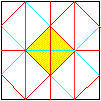Bascetta Star on the Internet        top

Deutsch

Annerose Gemeinhardt
Bascetta Stern   (.pdf-Datei)

Stephanie Meschke
3d-Stern, Sonobe-Stern  (.pdf Dateien)

Englisch

Jo Nakashima
Origami Bascetta Star  (Video)

Origami-Resource-Center.com
Sonobe Stellated Octahedron (12 units)

Feedback: Email address on my main page